# 5.5.2 BP/RP flux excess

Author(s): Carme Jordi, Claus Fabricius

To a first approximation we expect the sum of $BP$ and $RP$ fluxes to exceed the $G$ flux by only a small factor (due to the shapes of the passbands, see Figure 5.9) with a small dependence on colour.

The $BP$ and $RP$ fluxes, $I_{BP}$ and $I_{RP}$, are calculated as the sum of the flux in a window of $3.5\times 2.1$ arcsec${}^{2}$. Since the data processing for Gaia DR2 does not include deblending treatment, $I_{BP}$ and $I_{RP}$ can suffer contamination by nearby sources. Instead, the $G$ flux $I_{G}$ is derived from LSF or PSF fitting to a narrow image and the contamination issue is not significant. Any error in the background estimate also affects the $BP$ and $RP$ fluxes more than the $G$ flux. Therefore, if accurate colour information is required, the consistency of the three fluxes should be checked.

Figure 5.26 shows the median simple flux ratio $(I_{BP}+I_{RP})/I_{G}$ in galactic coordinates for the sources brighter and fainter than $G=18.5$. This ratio is called phot_bp_rp_excess_factor. One can appreciate that the faint sources have flux ratios larger than the bright set. One can also notice that the excess factor is larger in the ecliptic plane area indicating an under-subtraction of the sky background.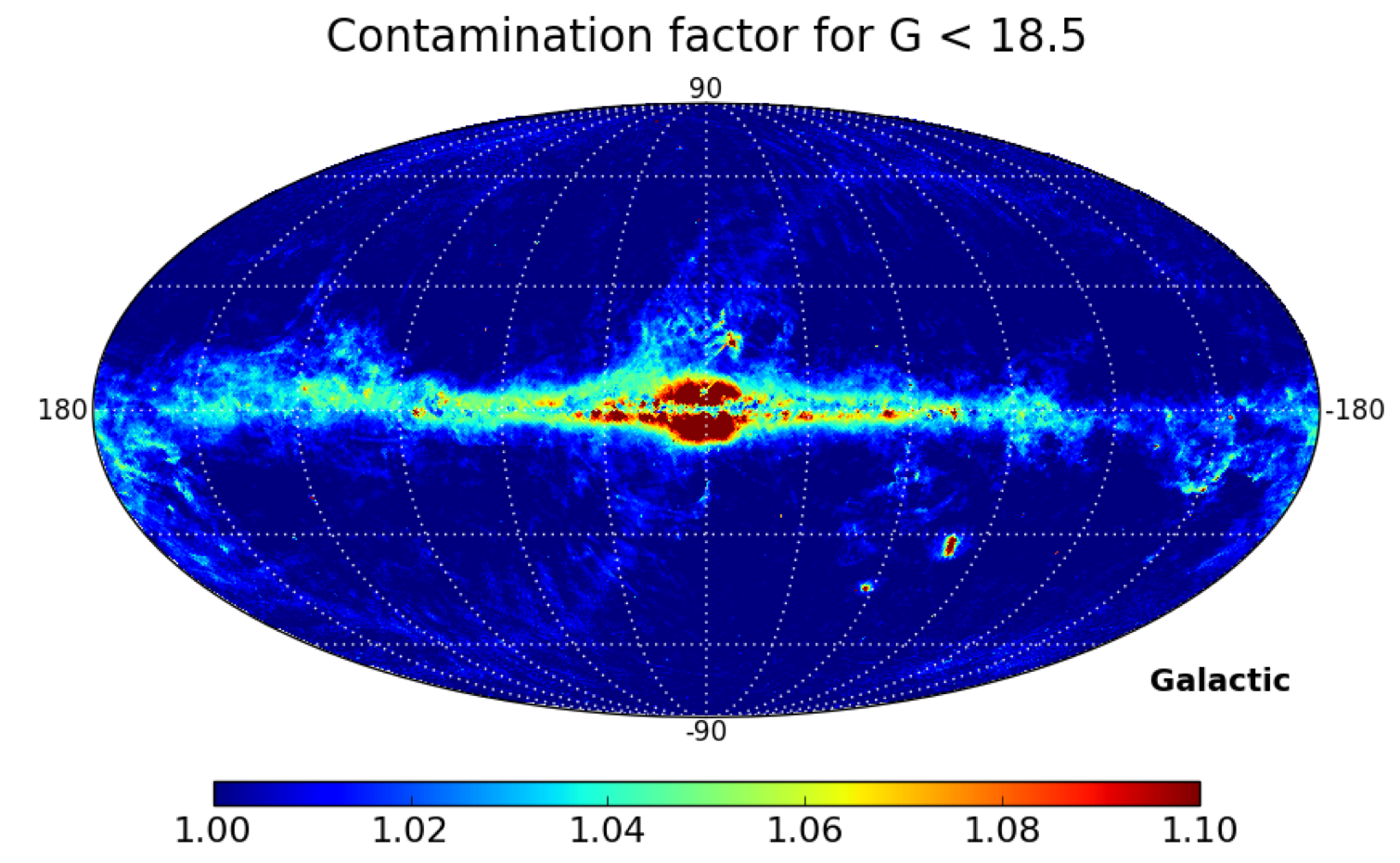Figure 5.26: Median flux ratio in galactic coordinates for random sets of sources brighter and fainter than G=18.5.

Figures 5.27 to  5.32 show the flux ratio (I${}_{G_{\rm BP}}$+I${}_{G_{\rm RP}}$)/I${}_{G}$ for several sets of sources: at high galactic latitudes, nearby sources, at the Galactic centre, of a globular cluster, in the sky area of LMC and quasars. The nearby sources, the QSOs and the sources at high latitudes are mainly affected by issues in the background subtraction. Sources in crowded areas like the Galactic centre, LMC area or globular clusters are mainly affected by contamination due to nearby sources.Figure 5.27: Excess factor for sources at high latitude. The plot at right is a zoom. The figures have been plotted before the filter at excess_factor=5 was applied. The dashed lines correspond to the relation 1.3+0.06⁢(GB⁢P-GR⁢P)2 and 1.0+0.015⁢(GB⁢P-GR⁢P)2 delimiting the band of well-behaved single sources.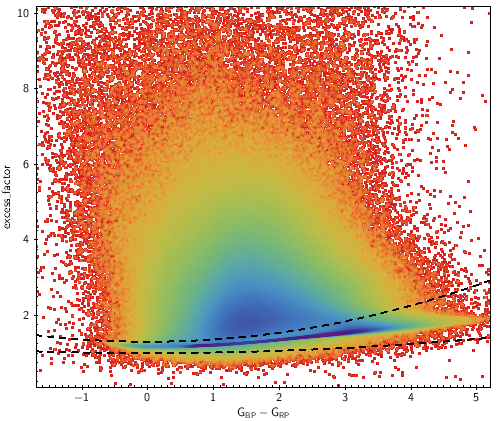Figure 5.28: As Fig. 5.27 now for the sources with ϖ>5 mas.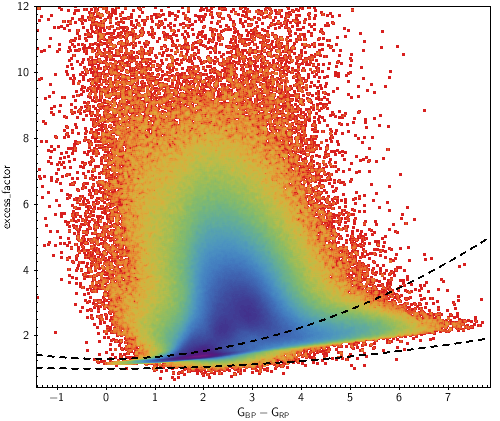Figure 5.29: As Fig. 5.27 now for the sources at the Galactic center, l=(-2o,2o) and b=(-2o,2o).Figure 5.30: As Fig. 5.27 now for the sources in a radius of 1 deg centered on 47 Tuc.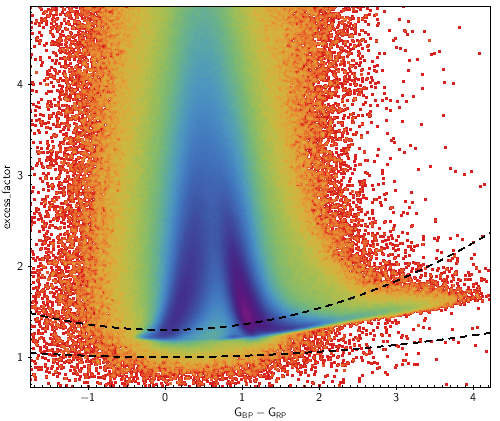Figure 5.31: As Fig. 5.27 now for the sources in the LMC sky area. Left: the sources in the bar. Right: the sources in the outskirt.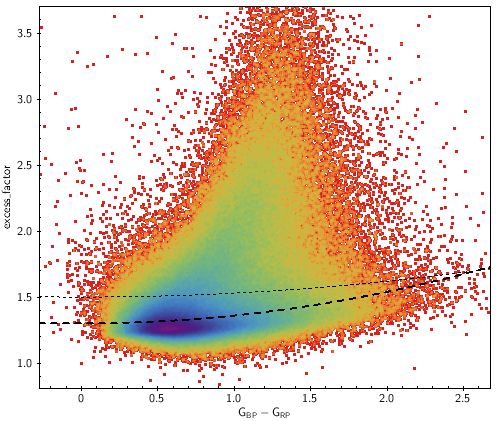Figure 5.32: As Fig. 5.27 now for a subset of QSOs. Dotted line corresponds to 1.5+0.03⁢(GB⁢P-GR⁢P)2 which seems more adequate than the threshold established with stars.

Figure 5.33 shows the case of sources close to a very bright star. The example shows the case of Sirius, but the same effect happens around any other bright star. There is a strong dependence of the excess factor with the distance to a bright star. The closer and fainter the source, the bluer it becomes deviating its position in the colour magnitude diagram.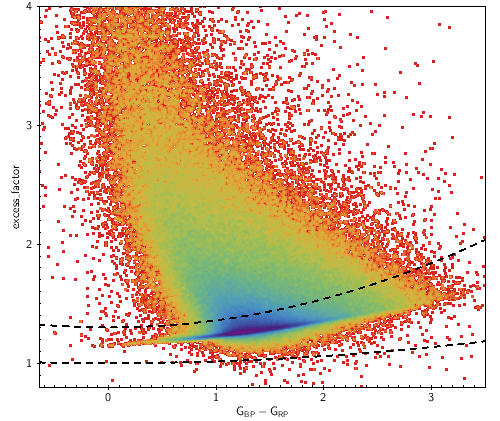Figure 5.33: The excess factor for the sources in a radius of 1.5 deg centered on Sirius. In the top left panel the colour code shows the density, while in the top right panel the colour code shows the angular distance to Sirius. The bottom left panel is the colour-magnitude diagram for the same sources and the bottom right panel shows the excess factor as a function of sky position. One can notice the strong dependence of the excess factor with the distance to Sirius. The closer to Sirius the bluer the source deviating its position in the colour magnitude diagram.

Figures 5.34 shows how well-behaved sources are shifted in the excess factor vs colour diagram when a contamination is added. The purpose of this simple simulation is to illustrate that (a) the $G_{\mathrm{BP}}-G_{\mathrm{RP}}$ colour does not change much when the contaminant source and the contaminated source have the same colour, (b) the red sources become bluer when the contaminant source is of blue colour, and (c) the other way around, the blue sources become redder when the contaminant source is of red colour.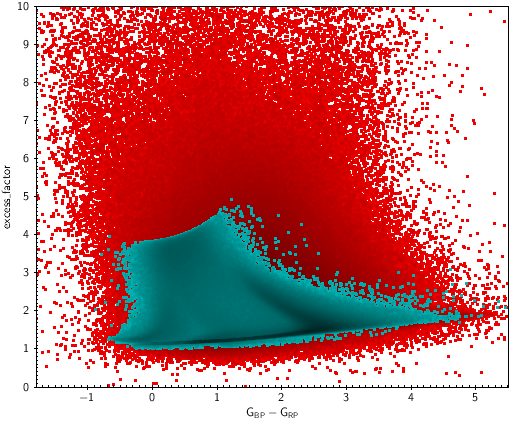Figure 5.34: Red dots are the nearby sources in Fig. 5.28. The blue dots show how well-behaved sources are shifted in the excess factor vs colour diagram factor when a contamination is added: a blue star of G=20 (top left), a red star of G=20 (top right), a blue star of G=17.5 (bottom left) and a red star of G=17.5 (bottom right).